# How to Sew a Half-Rectangle Triangle in Any Size

Half-rectangle triangles may seem just like half-square triangles, but they’re not quite the same. Half-rectangle triangles require a little more planning, but once you get the hang of it, you can use these shapes to bring a whole new design element to your quilting.

## What is a half-rectangle triangle?

A half-triangle rectangle is just that: an elongated triangle that forms half a rectangle. This shape can be used to make diamonds, zig zags and other interesting quilt designs.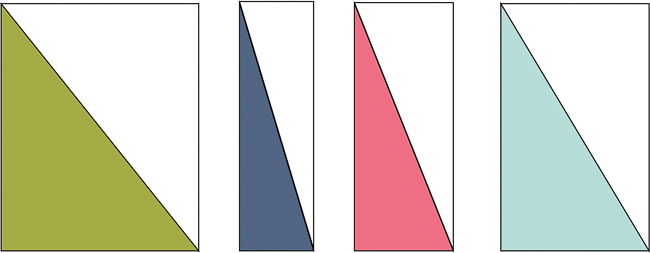## How to construct half-rectangle triangle units

Most quilters’ first instinct is to make a half-triangle rectangle the same way they make half-square triangles.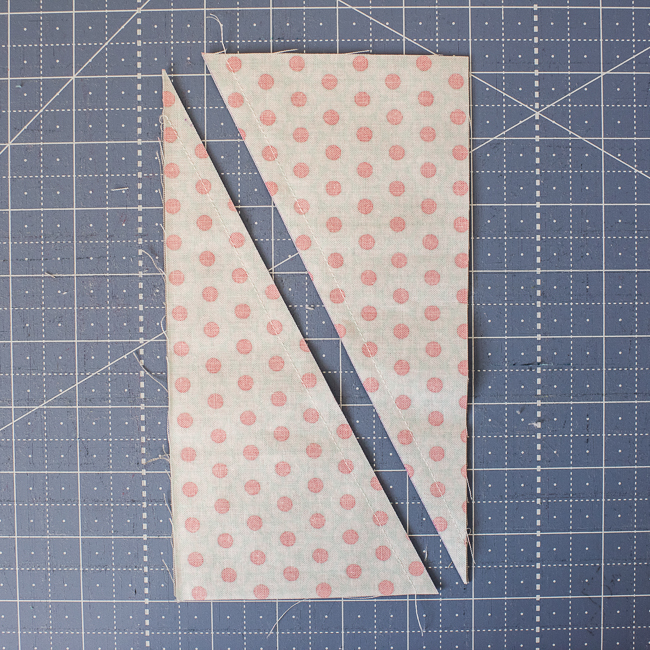But I’ll let you in on a secret: This doesn’t work! You can see below that you end up with an odd kite shape.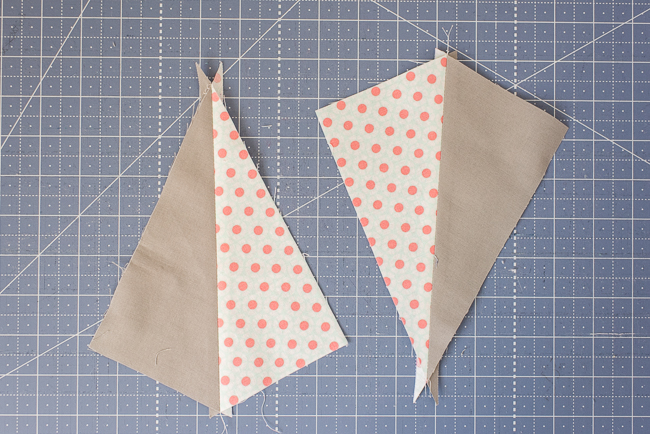A little more planning goes into sewing a half-triangle rectangle.

## Guidelines for sewing half-rectangle triangles

There isn’t a hard and fast formula to make half-rectangle triangles, but there are guidelines you can follow.

Start with the same size rectangle you want to finish with, and then add ¼” to the width and ½” to the length.

So, if you want the resulting half-rectangle triangle to be 3½” x 7½”, start with two 3¾” x 8″ rectangles. These half-triangle rectangles would finish at 3½” x 7½”.

### Easy half-rectangle triangles tutorial

I recommend using rectangles that have a 1:2 ratio for the length and width. A 1:2 ratio will result in a wider half-triangle rectangle rather than a long skinny shape. As the rectangle gets skinnier, it gets more difficult to work with.

This tutorial doesn’t require you to trim your half-triangle rectangles. If your units are coming out too small, increase the size a bit and then trim back down to the proper size.

### Step 1: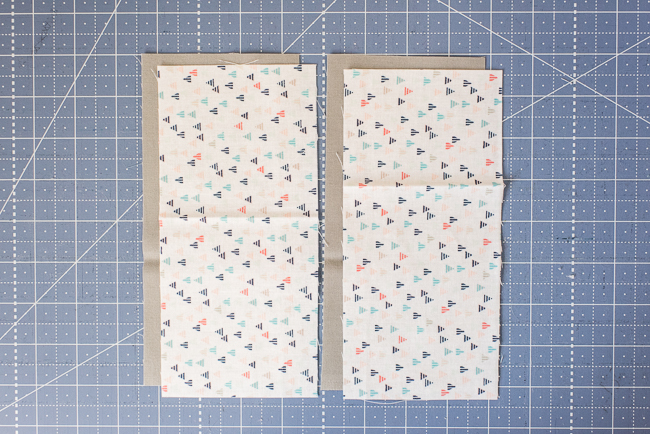Stack the two contrasting rectangles with all fabrics right side up.

### Step 2: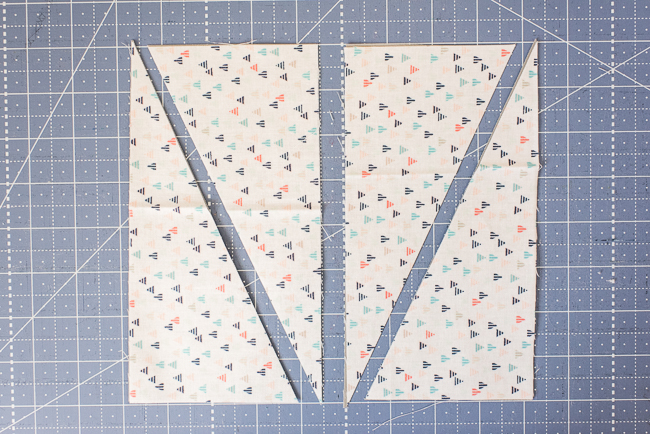Cut each rectangle on the diagonal. Keep in mind that each diagonal will make a half-rectangle triangle that goes in one direction. In this case, I’m cutting each pair of rectangles in the opposite directions.

### Step 3:

Sew the cut triangles back together, using two different fabrics and leaving a ¼” overlap on each side. Use a ¼”seam allowance.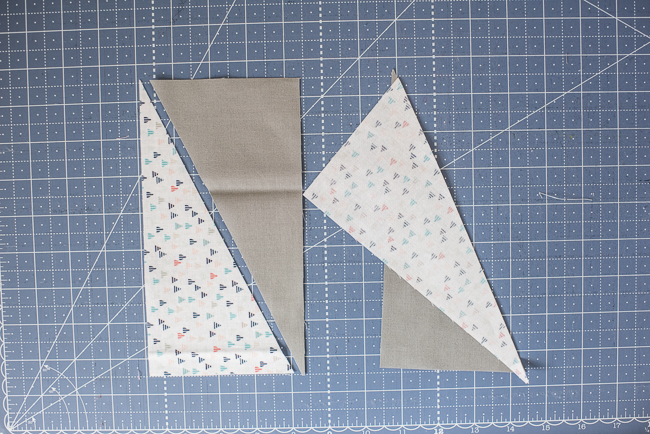Tip: Use starch to prevent the bias edges from stretching.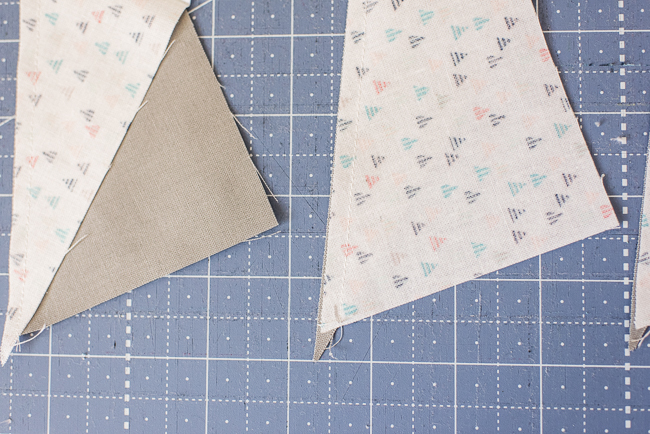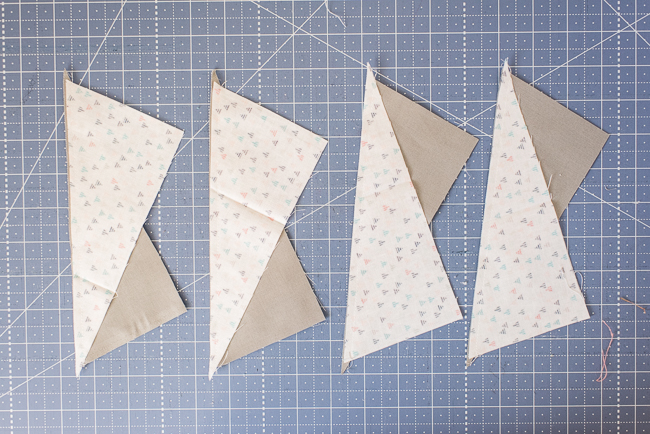### Step 4: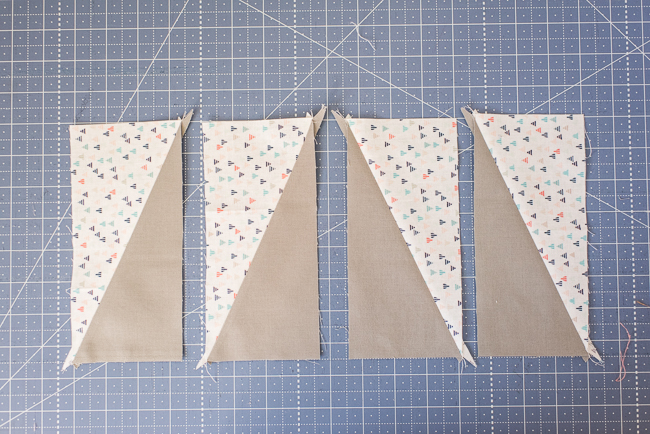Press the half-rectangle triangles open. Each pair of half-rectangle triangles is going in the opposite direction.

Four of these half-rectangle triangles make an elongated diamond. You can easily repeat these steps to make multiple diamonds or other shapes.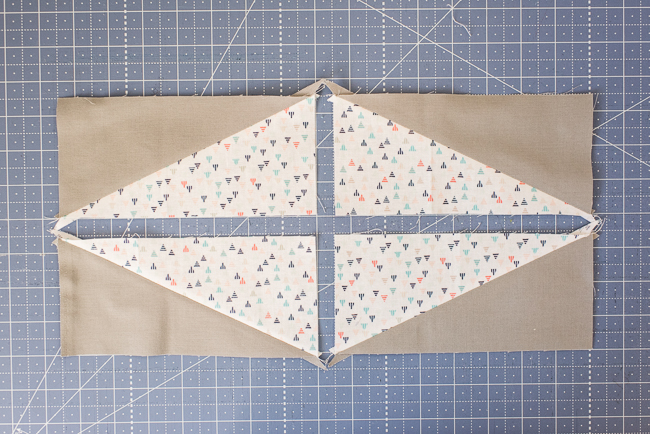## Make any size half-triangle rectangle

This same method works to make just about any size half-rectangle triangle. Below is a skinnier rectangle. These starting rectangles are 2¾” x 8″. The resulting half-triangle rectangles are 2½” x 8″.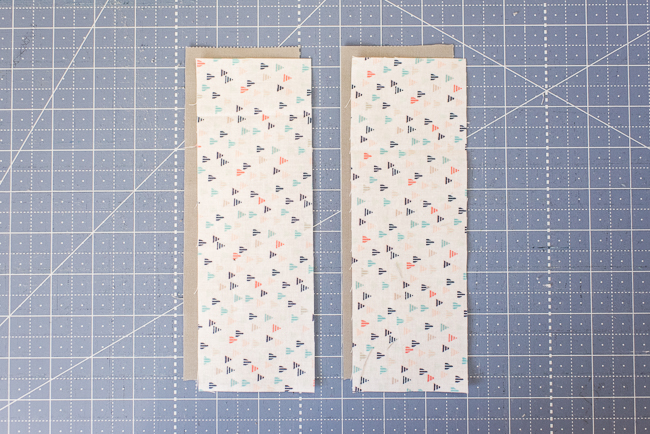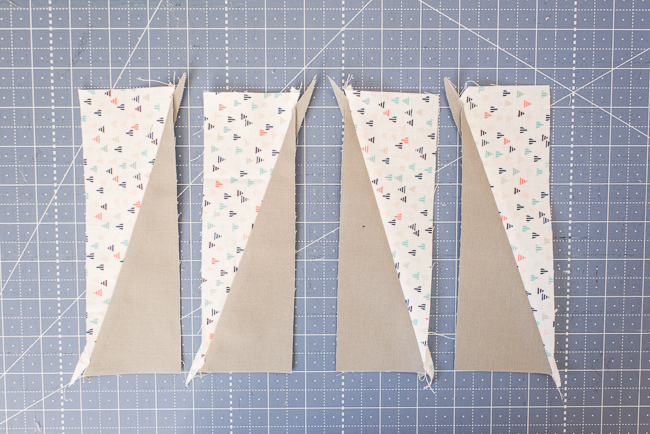Now we have two diamond shapes the same height but different widths. Experiment with different size starting rectangles to get various half-rectangle triangles.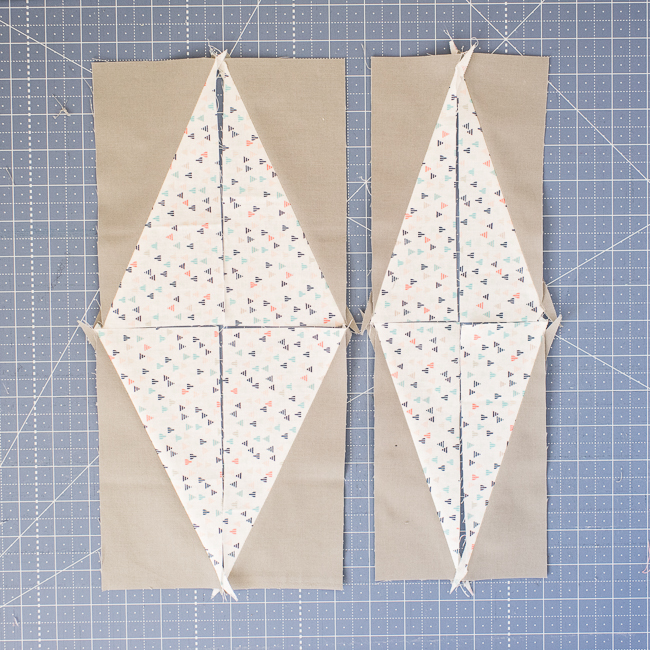## A common problem for diamond units

My first instinct was to sew the half-rectangle triangles together below to make diamond blocks. The side seam allowances kept coming up short, as you can see below.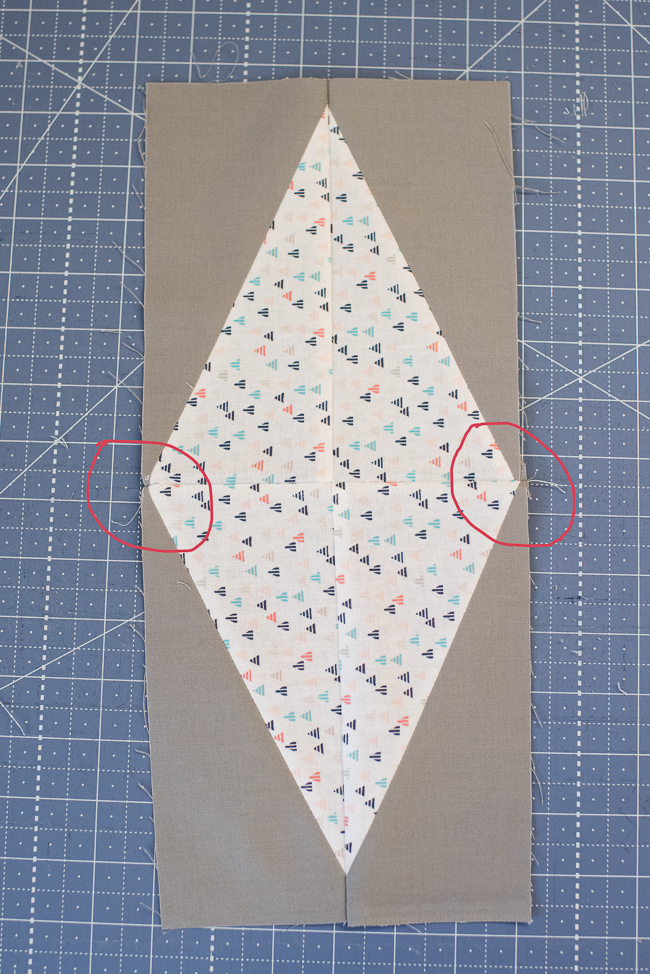A simple solution to this seam allowance problem is to use a bigger seam allowance. To create a diamond shape, first sew the top two half-triangle rectangles together.  Then sew the bottom two half-triangle rectangles together.

Now comes the step where the seam allowance will change.  Use a 3/8″ or ½” seam allowance when sewing the top and bottom units together.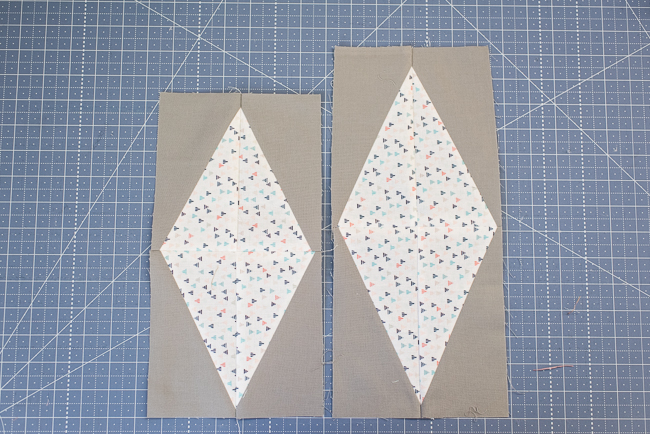Now that you know how to sew half-rectangle triangles, you can start dreaming up new quilt designs using this shape!